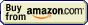# Iodometry## Potentiometric titration » Standard substances

Remember to use analytical reagents (AR grade) for standards.

Sodium oxalate Na2C2O4 - dry at 105-110°C. Equivalent weight is one-half the formula weight (133.9985 g).

Arsenic oxide As2O3 - dry for 2 hours at 105-110°C. Equivalent weight is one-fourth the formula weight (197.8414 g).

Potassium iodate KIO3 - dry for 2 hours at 150°C. Equivalent weight is one-sixth the formula weight (214.001 g).